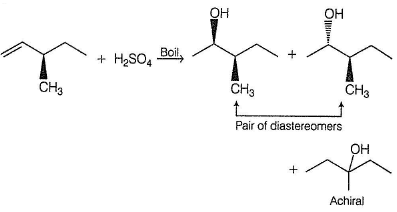Courses

# Test: Preparation Of Alcohols

## 22 Questions MCQ Test Chemistry for JEE | Test: Preparation Of Alcohols

Description
This mock test of Test: Preparation Of Alcohols for JEE helps you for every JEE entrance exam. This contains 22 Multiple Choice Questions for JEE Test: Preparation Of Alcohols (mcq) to study with solutions a complete question bank. The solved questions answers in this Test: Preparation Of Alcohols quiz give you a good mix of easy questions and tough questions. JEE students definitely take this Test: Preparation Of Alcohols exercise for a better result in the exam. You can find other Test: Preparation Of Alcohols extra questions, long questions & short questions for JEE on EduRev as well by searching above.
QUESTION: 1

### Direction (Q. Nos. 1-8) This section contains 8 multiple choice questions. Each question has four choices (a), (b), (c) and (d), out of which ONLY ONE is correct. Q,  Which of the following pairs of compounds can be used as starting material in the synthesis of 2-phenyl-2-pentanol?

Solution: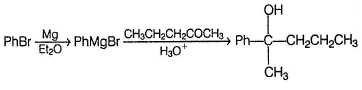QUESTION: 2

### What is the product of the reaction of methyl cyclohexene with B2H6 in THF followed by the oxidation with alkaline H2O2?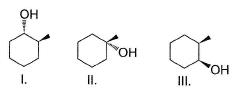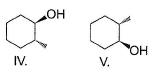Solution: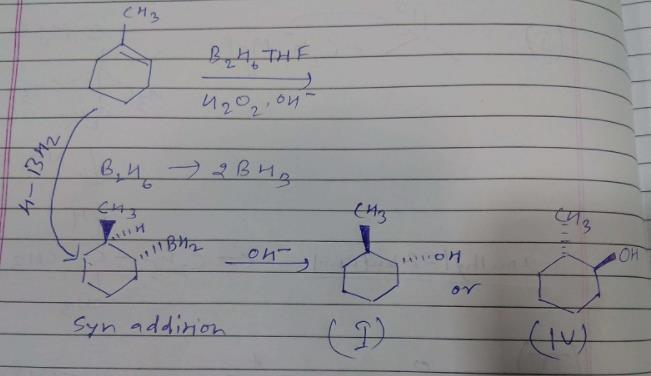QUESTION: 3

### Choose the reagent and reactant that would produce 2-methyl-2-butanol as the major product.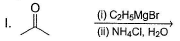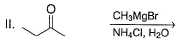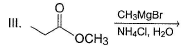Solution: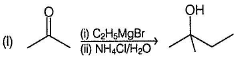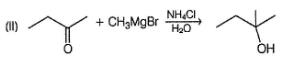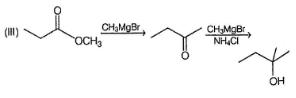QUESTION: 4

Ethanol is converted into ethoxy ethane,

Solution:

In order to obtain ethoxy ethane from ethanol, the ethanol must be subjected to the effect of concentrated sulphuric acid at 413 Kelvin or 140°C as shown below: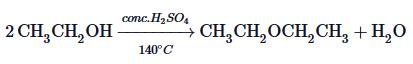QUESTION: 5

What is the correct structure for the major compound produced by the following reaction sequence?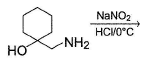Solution:

Reaction involves rearrangement of carbocation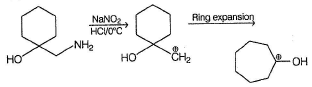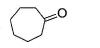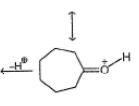QUESTION: 6

In the reaction given below,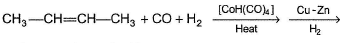The final major organic product is

Solution:

It is an oxo process of synthesis of alcohols.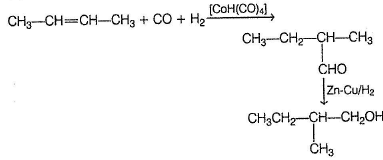QUESTION: 7

Primary alcohol can easily be prepared from primary alkyl halide via SN2 reaction with aqueous NaOH. However, similar method does not work for the preparation of tertiary alcohol. Which reaction can be used for the efficient preparation of tertiary alcohol {tertiary butanol) from tertiary butyl bromide?

Solution: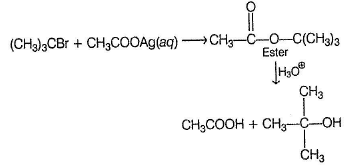QUESTION: 8

What is the major product in the following reaction?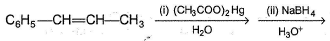Solution:

In oxymercuration-demercuration reaction, Markownikoff's addition of H2O takes place with HO- added to carbon where more stable carbocation could be formed.

*Multiple options can be correct
QUESTION: 9

Direction (Q. Nos. 9-15) This section contains 7 multiple choice questions. Each question has four choices (a), (b), (c) and (d), out of which ONE or MORE THAN ONE are correct.

In the following reaction,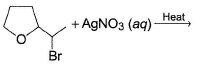The possible substitution product(s) is/are

Solution:

It is a SN1 reaction.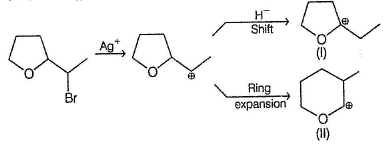(I) and (II) undergo nucleophilic attack by H2O giving the desired products.

*Multiple options can be correct
QUESTION: 10

In the following reaction,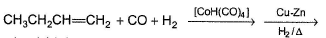Possible product(s) is/are

Solution:

Oxo proces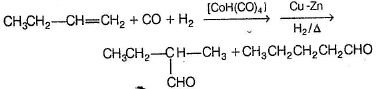The aldehydes formed above undergo reduction to give the desired products.

*Multiple options can be correct
QUESTION: 11

Which of the following Grignard’s synthesis can result into 2-cyclopentyl-2-butanol?

Solution:

Option (b) does not give 2-cyclopentyl-2-butanol as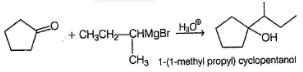*Multiple options can be correct
QUESTION: 12

Aliphatic alcohol when treated with dilute H2SO4, undergo isomerisation via reversible reaction. In the following reaction, which of the isomers are expected to be present at equilibrium?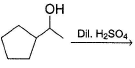Solution:

Isomerisation occur via carbocation intermediates.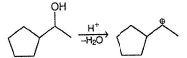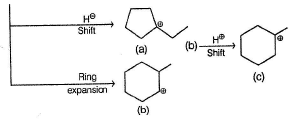The above carbocations upon nucleophilic attack by water gives the desired product.

*Multiple options can be correct
QUESTION: 13

In the following reaction,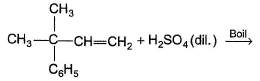The alcohol(s) formed in significant yield is/are

Solution: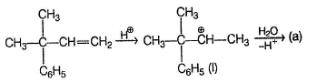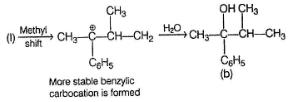*Multiple options can be correct
QUESTION: 14

In the reaction given below,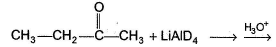The correct statement regarding the outcome of the above reaction is/are

Solution:

Deutride (D-) addition at planar carbonyl carbon occur from both side of plane, with equal probability giving racemic mixture of alcohols. Also deuterium is attached to carbonyl carbon atom only.

*Multiple options can be correct
QUESTION: 15

Consider the following reaction,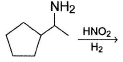Possible product(s) is/are

Solution:

Reaction proceed via carbocation intermediates.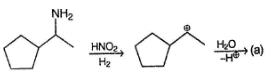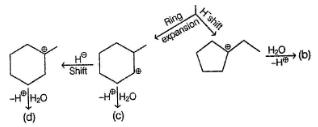QUESTION: 16

Comprehension Type

Direction (Q. Nos. 16-18) This section contains a paragraph, describing theory, experiments, data, etc.
Three questions related to the paragraph have been given. Each question has only one correct answer among the four given options (a), (b), (c) and (d).

Passage

Consider the following sequence of reaction,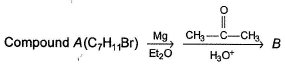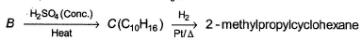Q.

The structure of compound B is

Solution:

B is an alcohol formed by the attack of Grignard's reagent on acetone. Hence, alcohol must have the skeleton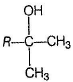Also, R is C7H11 with two degree of unsaturation it must be cyclohexenyl methyl not cyclohexyl methyl.

QUESTION: 17

Consider the following sequence of reaction,Q.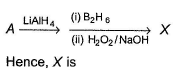Solution:

Hydroboration oxidation at double bond gives anti-Markownikoff’s addition of H2O in syn orientation.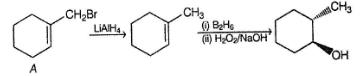QUESTION: 18

Consider the following sequence of reaction,Q.

If C is treated with excess of Br2(l) how many different isomers of bromination product(s) result?

Solution: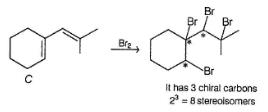*Answer can only contain numeric values
QUESTION: 19

One Integer Value Correct Type

Direction (Q. Nos. 19-22) This section contains 4 questions. When worked out will result in an integer from 0 to 9 (both inclusive).

In the following reaction,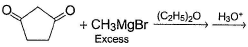Q.

How many different diols are formed as a result of nucleophilic addition reaction?

Solution: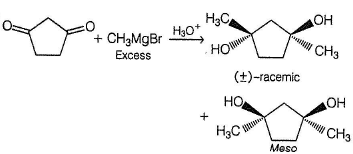*Answer can only contain numeric values
QUESTION: 20

In the reaction given below,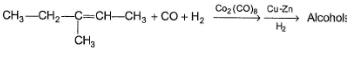How many different products are expected?

Solution: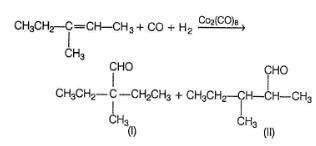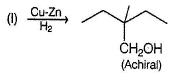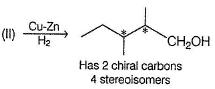Hence 5 is the correct answer.

*Answer can only contain numeric values
QUESTION: 21

If 3, 3-dimethyl-2, 4-pentanedione is treated with a Grignard reagent consisting of mixture of CH3MgBr and C2H5MgBr and finally hydrolysing product with dilute H2SO4 results in the formation of how many different diols?

Solution: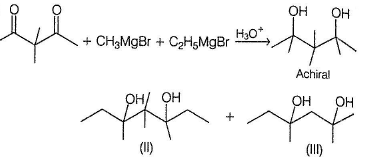(II) has two chiral carbon but symmetrical, hence, three stereoisomers.
(III) has only one chiral carbon hence, two stereoisomers (pair of enantiomers).

*Answer can only contain numeric values
QUESTION: 22

If a pure enantiomer of 3-methyl-1-pentene is treated with boiling solution of dilute H2SO4, how many different alcohols are expected in principle?

Solution: